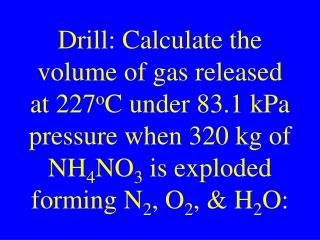DownloadDownload PresentationThermo-chemistry

# Thermo-chemistry

Télécharger la présentation## Thermo-chemistry

- - - - - - - - - - - - - - - - - - - - - - - - - - - E N D - - - - - - - - - - - - - - - - - - - - - - - - - - -
##### Presentation Transcript

1. Drill: Calculate the volume of gas released at 227oC under 83.1 kPa pressure when 320 kg of NH4NO3 is exploded forming N2, O2, & H2O:

2. Thermo-chemistry

3. AP Chm Chapter 8

4. Chm II Chapter 15

5. Thermochemistry • The study of heat transfer in chemical reactions

6. Thermochemistry Topics • Heat change • Calorimetry • Thermo-Chm-Eq

7. Thermo-chemical Terms

8. Heat (H) • A form of energy that can flow between samples of matter

9. Enthalpy • Another name for heat H

10. Enthalpy Change • Heat that can flow in/out of a system DH

11. System • That part of nature upon which attention is focused

12. Surroundings • That part of nature around the part upon which we are focused

13. Reaction Coordinate • A graph of energy change versus time in a chemical reaction

14. P Energy R Time

15. Exothermic Rxn • Chemical reactions that release, give off heat, or lose heat

16. Exothermic Rxn • Products will contain less heat than the reactants DH < 0

17. Endothermic Rxn • Chemical reactions that absorb, take in heat, or gain heat

18. Endothermic Rxn • Products will contain more heat than the reactants DH > 0

19. Topic: Heat Change

20. Specific Heat • The heat required to raise one gram of a substance 1oC • C: (J/goC, J/kgoK)

21. Specific Heat Formula DH = mCDT

22. Heat of Fusion • The heat required to melt one gram of a substance at its normal MP • Hf: (J/g or J/kg)

23. Heat of Fusion Formula DH = mHf

24. Heat of Vaporization • The heat required to boil one gram of a substance at its normal BP • Hv: (J/g or J/kg)

25. Heat of Vap. Formula DH = mHv

26. AP CHM HW • Read: Chapter 8 • Work problems: 5 & 17 • Page: 234

27. CHM II HW • Read: Chapter 9 • Work problems: • Page:

28. Calculate the heat change when 10.0 g H2O goes from –100.0oC to 200oC.MP = 0oC BP = 100oCHf = 334 J/g HV = 2260 J/g Cice = 2.06 J/gK Cwater = 4.18 J/gK Csteam = 2.02 J/gK

29. Drill: Calculate the heat required to change 25 g of water from 140.0oC to 60.0oC MP = 0oC BP = 100oCHf = 334 J/g HV = 2260 J/g Cice = 2.06 J/gK Cwater = 4.18 J/gK Csteam = 2.02 J/gK

30. Topic: Calorimetry

31. Calorimetry • A method to measure the experimental heat transfer in a system

32. Calorimeter • Device used to measure heat transfer • A calorimeter is an adiabatic system • Experimental yield

33. Adiabatic System • A system that exchanges zero heat with its surroundings DHsystem = 0

34. DH (J or kJ) DH = q = the heat or enthalpy change in a system DHsys = mcDTsysparts

35. Calorimetry DHsystem = 0 DHsys = DHcal + DHrxn DHrxn = -DHcal DHrxn = -mcDTcal

36. When Q reacts in a 1.5 kg calorimeter containing 2.5 kg water the temp changes from 22.5oC to 26.5oC.Calculate DHrxn. Cwater = 4.18 J/gK Ccal = 2.00 J/gK

37. Chm II HW Problems: 64 & 65 Page: 641-641

38. AP Chm HW Problems: 8 & 9 Page: 234

39. When X reacts in a 2.0 kg calorimeter containing 1.5 kg water, the temp changes from 22.5oC to 30.5oC. Calculate DHrxn. Cwater = 4.18 J/gK Ccal = 1.50 J/gK

40. When Z reacts in a 1.0 kg calorimeter containing 2.0 kg water, DT = 22.5oC to 32.5oC. Calculate DHrxn. Cwater = 4.18 J/gK Ccal = 2.00 J/gK

41. Drill: When 40.0 g NH4NO3 dissolves in 460.0 g water at 25.0oC, the temp. falls to 22.5.oC. Calculate the DHosoln for NH4NO3. Csoln = 4.00 J/gK

42. Topic: Thermochemical Equation (TCE)

43. Thermo-chemical Equation Terms

44. Heat of Reaction • The heat or enthalpy change of a chemical reaction DHrxn

45. Typical Reaction • HCl + NaOH  NaCl + HOH

46. Heat of Solution • The heat or enthalpy change when a substance is dissolved DHsoln

47. Typical Ionization KCl  K+(aq) + Cl-(aq)

48. Heat of Combustion • The heat or enthalpy change when a substance is burned DHcombustion

49. Combustion Reaction • CxHy + O2 • CO2 + HOH